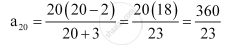CBSE (Arts) Class 11CBSE
Share
Notifications

View all notifications
Books Shortlist
Your shortlist is empty

# Find The 20th Term in the Following Sequence Whose Nth Term is A_N = (N(N-2))/(N+3) ;A_20 - CBSE (Arts) Class 11 - Mathematics

Login
Create free account

Forgot password?
ConceptConcept of Sequences

#### Question

Find the 20th term in the following sequence whose nth term is a_n = (n(n-2))/(n+3) ;a_20

#### Solution

Substituting = 20, we obtainIs there an error in this question or solution?

#### APPEARS IN

NCERT Solution for Mathematics Textbook for Class 11 (2018 to Current)
Chapter 9: Sequences and Series
Q: 10 | Page no. 180
Solution Find The 20th Term in the Following Sequence Whose Nth Term is A_N = (N(N-2))/(N+3) ;A_20 Concept: Concept of Sequences.
S